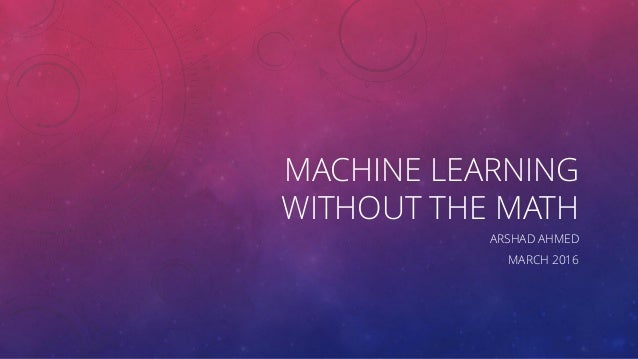Successfully reported this slideshow.Upcoming SlideShare
×

# Machine Learning without the Math: An overview of Machine Learning

359 views

Published on

A brief overview of Machine Learning and its associated tasks from a high level. This presentation discusses key concepts without the maths.The more mathematically inclined are referred to Bishops book on Pattern Recognition and Machine Learning.

Published in: Data & Analytics
• Full Name
Comment goes here.

Are you sure you want to Yes No• Be the first to comment

### Machine Learning without the Math: An overview of Machine Learning

1. 1. MACHINE LEARNING WITHOUT THE MATH ARSHAD AHMED MARCH 2016
2. 2. CONTENTS • What is machine learning? • Why do we need it? • Machine Learning Paradigms • Machine Learning Tasks • Desirable Properties • Common Problems • Summary and Conclusions
3. 3. WHAT IS MACHINE LEARNING? • Machine Learning is the study of computer algorithms that improve automatically through experience according to a performance measure • A lot of machine learning is a rediscovery of things that statisticians already knew. This is often disguised by differences in terminology: • Ridge regression = weight-decay • Fitting = learning • Held-out data = test data Why do this? “Instead of writing a program by hand, we collect lots of examples that specify the correct output for a given input. A machine learning algorithm then takes these examples and produces a program that does the job. This program may look very different from a typical hand-written program. It may contain millions of numbers. If we do it right, the program works for new cases as well as the ones we trained it on”. -- Geoffrey Hinton
4. 4. WHY DO WE NEED IT? • Simply put BIG DATA!
5. 5. MACHINE LEARNING PARADIGMS • Supervised Learning • Unsupervised Learning • Reinforcement Learning
6. 6. SUPERVISED LEARNING The algorithm is presented with training data which consists of examples which include both the inputs and the desired outputs, thus enabling it to learn a function. The learner should then be able to generalize from the presented data to unseen examples.
7. 7. UNSUPERVISED LEARNING The algorithm is presented with examples from the input space only and a model is fit to these observations. For example, a clustering algorithm would be a form of unsupervised learning.
8. 8. DEEP LEARNING EXAMPLE: CONVOLUTIONAL NEURAL NETWORKS (CONVNETS) A neural network method that is very powerful and works well with GPU computation paradigm. It is unsupervised because it does automatic feature extraction from raw input data.
9. 9. REINFORCEMENT LEARNING An agent explores an environment and at the end receives a reward, which may be either positive or negative. In effect, the agent is told whether he was right or wrong, but is not told how.
10. 10. A CLASSIC MACHINE LEARNING TASK
11. 11. OTHER MACHINE LEARNING TASKS • Pattern recognition • Medical Images • Facial Expressions • Spoken Word -> Digital Assistants • Pattern Generation • Generating images or motion sequences for example to fill a gap • Anomaly Detection • Network Intrusion Detection • Novelty Detection • Fraud Detection
12. 12. DESIRABLE PROPERTIES • Simple solutions are appropriately favoured over complicated ones. • Powerful enough to learn the solution to a given problem. • Stable to parameter variations. • Converges in finite time. • Scales reasonably with the number of training examples, the number of input features and the number of test examples.
13. 13. COMMON PROBLEMS • Overfitting / Under fitting • A trade off between complexity and good ness of fit of the model • Generalisation
14. 14. SUMMARY AND CONCLUSIONS • We defined machine learning as consisting of supervised and unsupervised tasks • The purpose of this is to avoid tedious programming tasks and have the machine learn from examples. • It is a powerful tool with wide applications but understanding of the limitations and evaluation techniques are very important • The goal of any machine learning is to have a model with high accuracy and generalizability
15. 15. THANK YOU FOR LISTENING QUESTIONS?